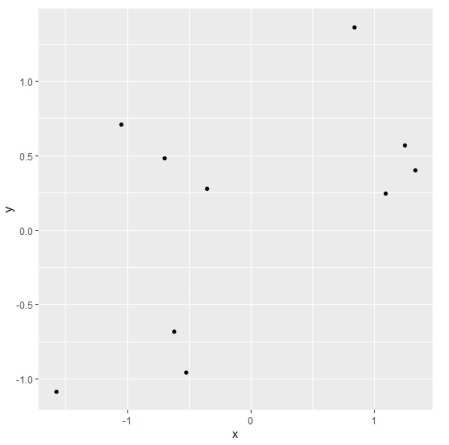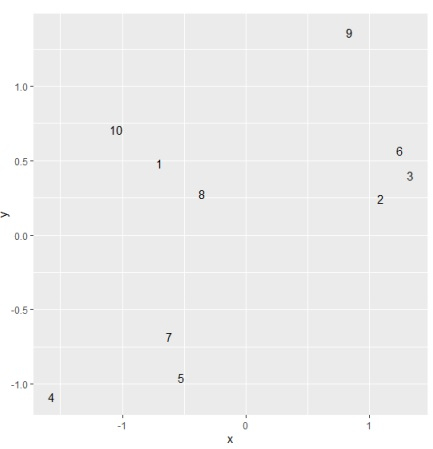# How to display ID column values in a scatterplot created with ggplot2 in R?

To display ID column values in a scatterplot created with ggplot2 in R, we can follow the below steps −

• First of all, create a data frame.
• Then, create the scatterplot using ggplot2.
• After that, create the same plot with label argument inside aes and add the geom_text function.

## Create the data frame

Let's create a data frame as shown below −

Live Demo

> ID<-1:10
> x<-rnorm(10)
> y<-rnorm(10)
> df<-data.frame(ID,x,y)
> df

On executing, the above script generates the below output(this output will vary on your system due to randomization) −

  ID     x         y
1 1   -0.6980655  0.4815529
2 2    1.0943027  0.2476090
3 3    1.3299122  0.4023055
4 4   -1.5713752 -1.0870443
5 5   -0.5254172 -0.9559608
6 6    1.2467670  0.5690500
7 7   -0.6193448 -0.6816401
8 8   -0.3558055  0.2776055
9 9    0.8396879  1.3638565
10 10 -1.0508085  0.7066900

## Create the scatterplot using ggplot2

Using geom_point function of ggplot2 package to create the scatterplot −

> ID<-1:10
> x<-rnorm(10)
> y<-rnorm(10)
> df<-data.frame(ID,x,y)
> library(ggplot2)
> ggplot(df,aes(x,y))+geom_point()

## Output## Create the scatterplot with ID column values displayed on the plot

Using geom_text function and use label argument inside aes of ggplot function to create the scatterplot with ID column values displayed on the plot −

> ID<-1:10
> x<-rnorm(10)
> y<-rnorm(10)
> df<-data.frame(ID,x,y)
> library(ggplot2)
> ggplot(df,aes(x,y,label=ID))+geom_text()

## Output